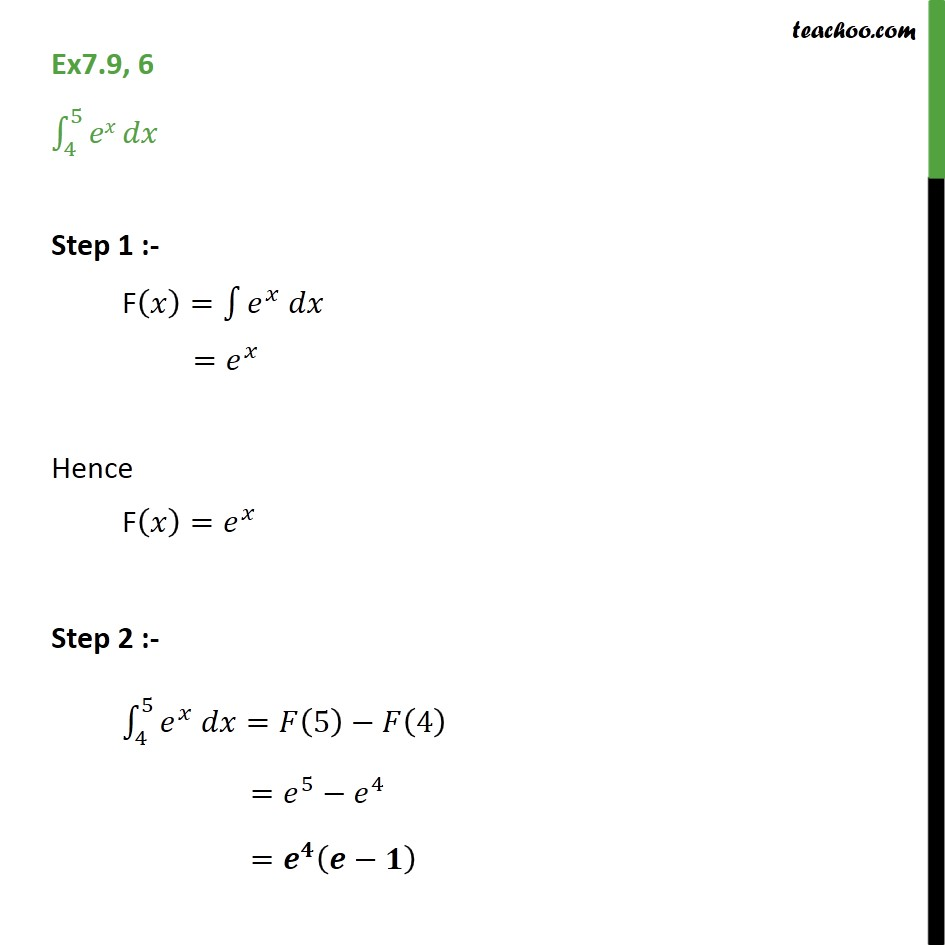Integration Full Chapter Explained - Integration Class 12 - Everything you need1. Chapter 7 Class 12 Integrals
2. Concept wise
3. Definite Integration - By Formulae

Transcript

Ex 7.9, 6 - Chapter 7 Class 12 Integration - Teachoo Direct Integrate ∫ 4 -> 5 e^x dx Step 1 :- F(x) = ∫ e^x dx = e^x Hence F(x) = e^x Step 2 :- ∫ 4 -> 5 e^x dx = F(5) - F(4) = e^5 - e^4 = e^4 (e - 1)

Definite Integration - By Formulae

About the AuthorDavneet Singh
Davneet Singh is a graduate from Indian Institute of Technology, Kanpur. He has been teaching from the past 9 years. He provides courses for Maths and Science at Teachoo.